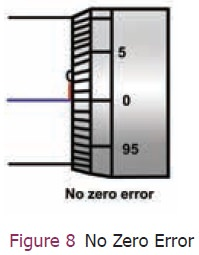Home | | Science 9th std | Screw Gauge

# Screw Gauge

Measurements made with a Vernier caliper can be made in centimetre only.

Screw Gauge

Measurements made with a Vernier caliper can be made in centimetre only. Hence to measure the length and thickness of very small objects we use a screw gauge. This instrument can measure the dimensions upto 1/100th of a millimetre or 0.01 mm. With the screw gauge it is possible to measure the diameter of a thin wire and the thickness of thin metallic plates.

## 1. Description of screw gauge

The screw gauge consists of a U shaped metal frame. A hollow cylinder is attached to one end of the frame. Grooves are cut on the inner surface of the cylinder through which a screw passes (Figure 7).On the cylinder parallel to the axis of the screw there is a scale which is graduated in millimetre called Pitch Scale (PS). One end of the screw is attached to a sleeve. The head of the sleeve (Thimble) is divided into 100 divisions called the Head scale.

The end of the screw has a plane surface (Spindle). A stud (Anvil) is attached to the other end of the frame, just opposite to the tip of the screw. The screw head is provided with a ratchat arrangement (safety device) to prevent the user from exerting undue pressure.## 2. Using the screw gauge

The screw gauge works on the principal that when a screw is rotated in a nut, the distance moved by the tip of the screw is directly proportional to the number of rotations.

### Pitch of the screw

The pitch of the screw is the distance between two successive screw threads. It is also equal to the distance travelled by the tip of the screw for one complete rotation of the head. It is equal to 1 mm in typical screw gauges.

Pitch of the screw = Distance moved by the Pitch / No. of rotations by Head scale

### Least count of a screw gauge

The distance moved by the tip of the screw for a rotation of one division on the head scale is called the least count of the screw gauge.

Least count of the instrument (L.C.) = Value of one smallest pitch scale reading / Total number of Head scale division

### Zero Error of a screw gauge

When the plane surface of the screw and the opposite plane stud on the frame area brought into contact, if the zero of the head scale coincides with the pitch scale axis there is no zero error (Figure 8).### Positive zero error

When the plane surface of the screw and the opposite plane stud on the frame are brought into contact, if the zero of the head scale lies below the pitch scale axis, the zero error is positive (Figure 9) . For example, the 5th division of the head scale coincides with the pitch scale axis, then the zero error is positive and is given by Z.E = + (n × LC) where ‘n’ is the head scale coincidence. In this case, Zero error + (5 × 0.01) = 0.05mm. So the zero correction is – 0.05 mm.### Negative zero error

When the plane surface of the screw and the opposite plane stud on the frame are brought into contact, if the zero of the head scale lies above the pitch scale axis, the zero error is negative (Figure 10). For example, the 95th division coincides with the pitch scale axis, then the zero error is negative and is given by

ZE = – (100 – n) × LC
ZE = – (100 – 95) × LC
= – 5 × 0.01
= – 0.05 mm
The zero correction is + 0.05mm.## 3. To measure the thicknessof a thin coin using a screw gauge

·        Determine the pitch, the least count and the zero error of the screw gauge
·        Place the coin between the two studs
·        Rotate the head until the coin is held firmly but not tightly, with the help of the ratchat
·        Note the reading of the pitch scale crossed by the head scale (PSR) and the head scale division that coincides with the pitch scale axis (HSC)
·        The width of the coin is given by PSR
·        CHSR (Corrected HSR). Repeat the experiment for different positions of the coin